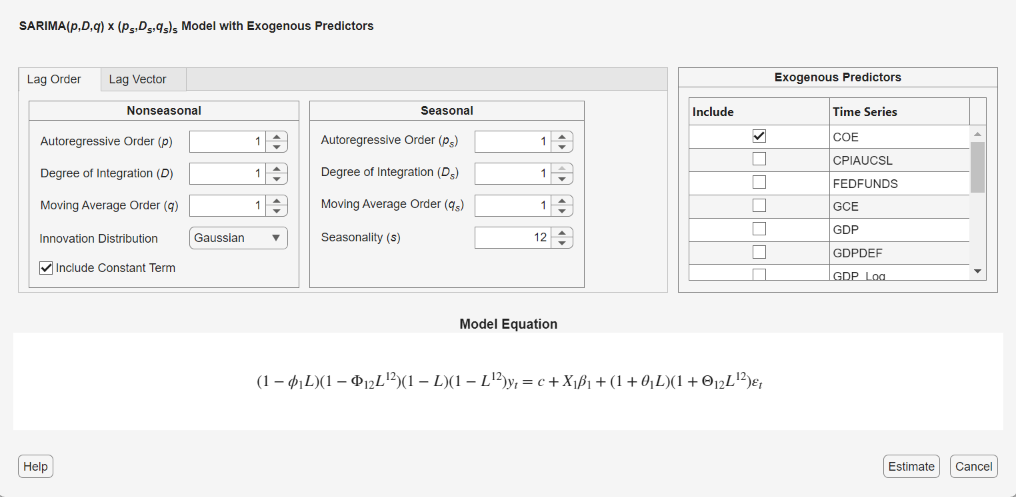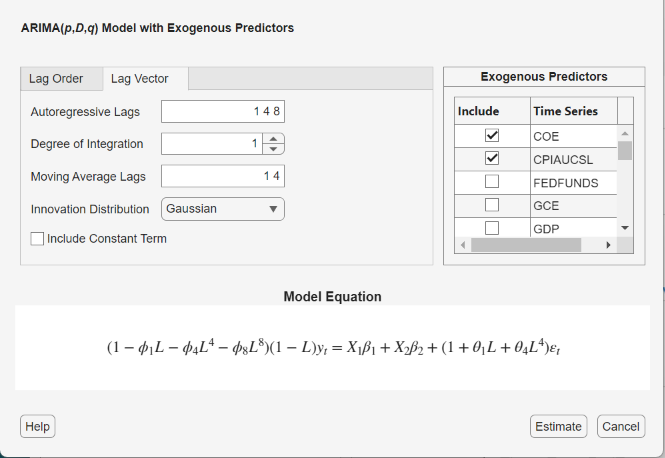## ARIMAX Model Specifications

### Create ARIMAX Model Using Name-Value Pairs

This example shows how to specify an ARIMAX model using `arima`.

Specify the ARIMAX(1,1,0) model that includes three predictors:

`$\left(1-0.1L\right)\left(1-L{\right)}^{1}{y}_{t}={x}_{t}^{\prime }{\left[\begin{array}{ccc}3& -2& 5\end{array}\right]}^{\prime }+{\epsilon }_{t}.$`

`Mdl = arima('AR',0.1,'D',1,'Beta',[3 -2 5])`
```Mdl = arima with properties: Description: "ARIMAX(1,1,0) Model (Gaussian Distribution)" Distribution: Name = "Gaussian" P: 2 D: 1 Q: 0 Constant: NaN AR: {0.1} at lag  SAR: {} MA: {} SMA: {} Seasonality: 0 Beta: [3 -2 5] Variance: NaN ```

The output shows that the ARIMAX model `Mdl` has the following qualities:

• Property `P` in the output is the sum of the autoregressive lags and the degree of integration, i.e., `P` = `p` + `D` = `2`.

• `Beta` contains three coefficients corresponding to the effect that the predictors have on the response. `Mdl` does not store predictor or response data. You specify the required data when you operate on `Mdl`.

• The rest of the properties are 0, `NaN`, or empty cells.

Be aware that if you specify nonzero `D` or `Seasonality`, then Econometrics Toolbox™ differences the response series ${y}_{t}$ before the predictors enter the model. Therefore, the predictors enter a stationary model with respect to the response series ${y}_{t}$. You should preprocess the predictors ${x}_{t}$ by testing for stationarity and differencing if any are unit root nonstationary. If any nonstationary predictor enters the model, then the false negative rate for significance tests of $\beta$ can increase.

### Specify ARMAX Model Using Dot Notation

This example shows how to specify a stationary ARMAX model using `arima`.

Specify the ARMAX(2,1) model

`${y}_{t}=6+0.2{y}_{t-1}-0.3{y}_{t-2}+3{x}_{t}+{\epsilon }_{t}+0.1{\epsilon }_{t-1}$`

by including one stationary exogenous covariate in `arima`.

` Mdl = arima('AR',[0.2 -0.3],'MA',0.1,'Constant',6,'Beta',3)`
```Mdl = arima with properties: Description: "ARIMAX(2,0,1) Model (Gaussian Distribution)" Distribution: Name = "Gaussian" P: 2 D: 0 Q: 1 Constant: 6 AR: {0.2 -0.3} at lags [1 2] SAR: {} MA: {0.1} at lag  SMA: {} Seasonality: 0 Beta:  Variance: NaN ```

The output shows the model `Mdl` has `NaN` values or an empty cell (`{}`) for the `Variance`, `SAR`, and `SMA` properties. You can modify the model using dot notation at the command line. For example, you can introduce another exogenous, stationary covariate, and specify the variance of the innovations as 0.1

`${y}_{t}=6+0.2{y}_{t-1}-0.3{y}_{t-2}+{x}_{t}^{\prime }\left[\begin{array}{c}3\\ -2\end{array}\right]+{\epsilon }_{t}+0.1{\epsilon }_{t-1};\phantom{\rule{0.2777777777777778em}{0ex}}\phantom{\rule{0.2777777777777778em}{0ex}}{\epsilon }_{t}\sim N\left(0,0.1\right).$`

Modify the model by specifying an innovations variance of 0.1 and regression coefficient values.

```Mdl.Beta = [3 -2]; Mdl.Variance = 0.1; Mdl```
```Mdl = arima with properties: Description: "ARIMAX(2,0,1) Model (Gaussian Distribution)" Distribution: Name = "Gaussian" P: 2 D: 0 Q: 1 Constant: 6 AR: {0.2 -0.3} at lags [1 2] SAR: {} MA: {0.1} at lag  SMA: {} Seasonality: 0 Beta: [3 -2] Variance: 0.1 ```

### Specify ARIMAX or SARIMAX Model Using Econometric Modeler App

In the Econometric Modeler app, you can specify the seasonal and nonseasonal lag structure, presence of a constant, innovation distribution, and predictor variables of an ARIMA(p,D,q) or a SARIMA(p,D,q)×(ps,Ds,qs)s model by following these steps. All specified coefficients are unknown but estimable parameters.

1. At the command line, open the Econometric Modeler app.

`econometricModeler`

Alternatively, open the app from the apps gallery (see Econometric Modeler).

2. In the Time Series pane, select the response time series to which the model will be fit.

3. On the Econometric Modeler tab, in the Models section, click the arrow to display the models gallery. For strictly nonseasonal models, click ARIMAX; for seasonal models, click SARIMAX. ARIMAX and SARIMAX models must contain at least one predictor variable.

The `Type` Model Parameters dialog box appears. This figure shows the SARIMAX Model Parameters dialog box. All variables in the Time Series pane, except the chosen response variable, appear in the Predictors section.4. Specify the lag structure. Use the Lag Order tab to specify a model that includes:

• All consecutive lags from 1 through their respective orders, in the seasonal polynomials

• Lags that are all consecutive multiples of the period (s), in the seasonal polynomials

• An s-degree seasonal integration polynomial

For the flexibility to specify the inclusion of particular lags, use the Lag Vector tab. For more details, see Specifying Univariate Lag Operator Polynomials Interactively. Regardless of the tab you use, you can verify the model form by inspecting the equation in the Model Equation section.

5. In the Predictors section, choose at least one predictor variable by selecting the Include? check box for the time series.

For example, suppose you are working with the `Data_USEconModel.mat` data set and its variables are listed in the Time Series pane.

• To specify an ARIMAX(3,1,2) model for the unemployment rate containing a constant, all consecutive AR and MA lags from 1 through their respective orders, Gaussian-distributed innovations, and the predictor variables COE, CPIAUCSL, FEDFUNDS, and GDP:

1. In the Time Series pane, select the `UNRATE` time series.

2. On the Econometric Modeler tab, in the Models section, click the arrow to display the models gallery.

3. In the models gallery, in the ARMA/ARIMA Models section, click .

4. In the ARIMAX Model Parameters dialog box in the Nonseasonal section of the Lag Order tab, set Degree of Integration to `1`.

5. Set Autoregressive Order to `3`.

6. Set Moving Average Order to `2`.

7. In the Predictors section, select the Include? check box for the COE, CPIAUCSL, FEDFUNDS, and GDP time series.

• To specify an ARIMAX(3,1,2) model for the unemployment rate containing all AR and MA lags from 1 through their respective orders, Gaussian-distributed innovations, no constant, and the predictor variables COE and CPIAUCSL:

1. In the Time Series pane, select the `UNRATE` time series.

2. On the Econometric Modeler tab, in the Models section, click the arrow to display the models gallery.

3. In the models gallery, in the ARMA/ARIMA Models section, click .

4. In the ARIMAX Model Parameters dialog box, in the Nonseasonal section of the Lag Order tab, set Degree of Integration to `1`.

5. Set Autoregressive Order to `3`.

6. Set Moving Average Order to `2`.

7. Clear the Include Constant Term check box.

8. In the Predictors section, select the Include? check box for the COE and CPIAUCSL time series.

• To specify an ARMA(8,1,4) model for the unemployment rate containing nonconsecutive lags

`$\left(1-{\varphi }_{1}L-{\varphi }_{4}{L}^{4}-{\varphi }_{8}{L}^{8}\right)\left(1-L\right){y}_{t}=\left(1+{\theta }_{1}L+{\theta }_{4}{L}^{4}\right){\epsilon }_{t}+{\beta }_{1}CO{E}_{t}+{\beta }_{2}CPIAUCS{L}_{t},$`

where εt is a series of IID Gaussian innovations:

1. In the Time Series pane, select the `UNRATE` time series.

2. On the Econometric Modeler tab, in the Models section, click the arrow to display the models gallery.

3. In the models gallery, in the ARMA/ARIMA Models section, click .

4. In the ARIMAX Model Parameters dialog box, click the Lag Vector tab.

5. Set Degree of Integration to `1`.

6. Set Autoregressive Lags to `1 4 8`.

7. Set Moving Average Lags to `1 4`.

8. Clear the Include Constant Term check box.

9. In the Predictors section, select the Include? check box for the COE and CPIAUCSL time series.• To specify an ARIMA(3,1,2) model for the unemployment rate containing all consecutive AR and MA lags through their respective orders, a constant term, the predictor variables COE and CPIAUCSL, and t-distributed innovations:

1. In the Time Series pane, select the `UNRATE` time series.

2. On the Econometric Modeler tab, in the Models section, click the arrow to display the models gallery.

3. In the models gallery, in the ARMA/ARIMA Models section, click .

4. In the ARIMAX Model Parameters dialog box, in the Nonseasonal section of the Lag Order tab, set Degree of Integration to `1`.

5. Set Autoregressive Order to `3`.

6. Set Moving Average Order to `2`.

7. Click the button, then select `t`.

8. In the Predictors section, select the Include? check box for COE and CPIAUCSL time series.

The degrees of freedom parameter of the t distribution is an unknown but estimable parameter.

After you specify a model, click to estimate all unknown parameters in the model.